# 监督学习和kNN分类初学者教程• 监督学习

• 了解数据

• kNN分类器模型

• 过拟合与欠拟合

• 结论

### 监督学习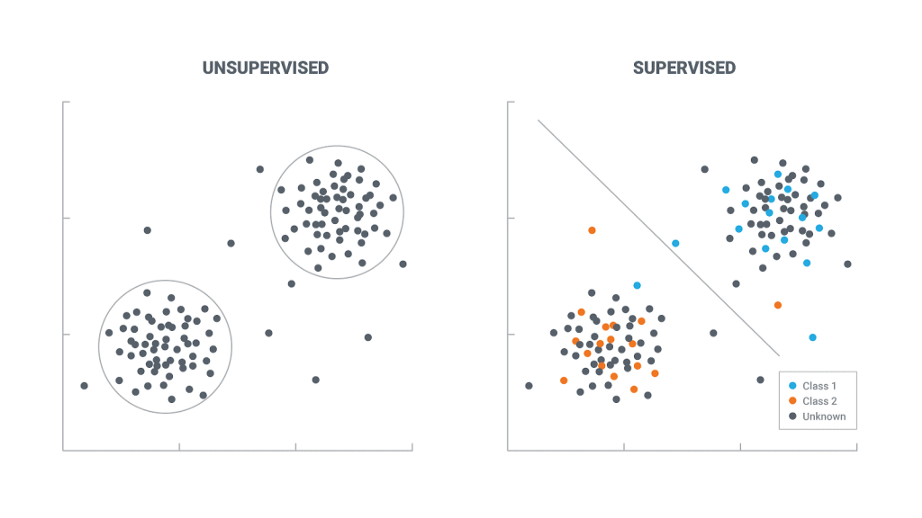### 库

#### 安装库

pip install scikit-learn

pip install numpy matplotlib

#### 导入库

from sklearn import datasets
from sklearn.neighbors import KNeighborsClassifier
from sklearn.model_selection import train_test_split

import matplotlib.pyplot as plt
import numpy as np

### 了解数据

digits = datasets.load_digits()

print(digits.keys)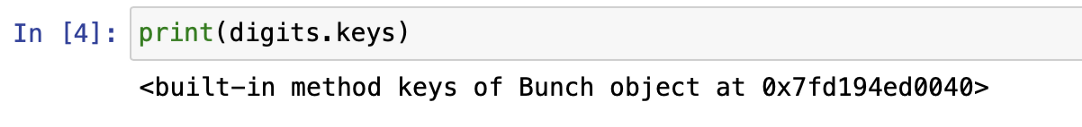Bunch是一个提供属性样式访问的Python字典。Bunch就像字典。

print(digits.DESCR)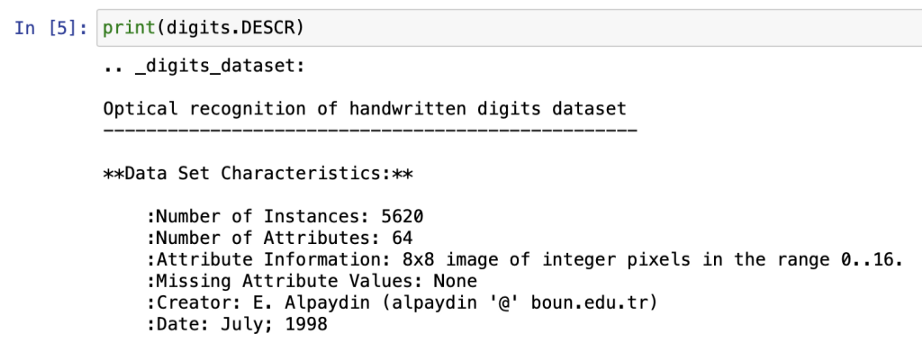plt.imshow(digits.images, cmap=plt.cm.gray_r, interpolation='nearest')
plt.show()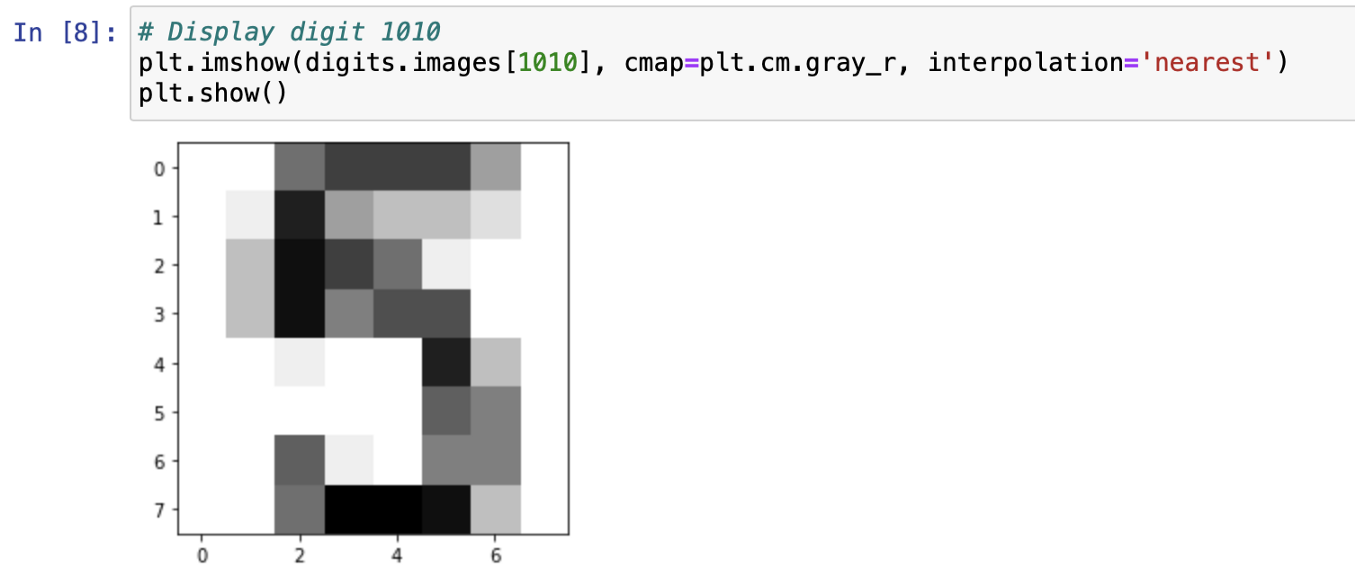### K近邻分类器

k-最近邻算法（k-NN）是一种用于分类和回归的非参数方法。在这两种情况下，输入由特征空间中k个最近的训练样本组成。输出取决于k-NN是用于分类还是回归。”（参考：https://en.wikipedia.org/wiki/K-nearest_neighbors_algorithm)

#### 特征和目标变量

X = digits.data
y = digits.target

#### 拆分数据

#test size 是指将数据集中作为测试数据的比率，其余将是训练数据

X_train, X_test, y_train, y_test = train_test_split(X, y, test_size = 0.2, random_state=42, stratify=y)

#### 定义分类器

knn = KNeighborsClassifier(n_neighbors = 7)

#### 拟合模型

knn.fit(X_train, y_train)

#### 准确度得分

print(knn.score(X_test, y_test))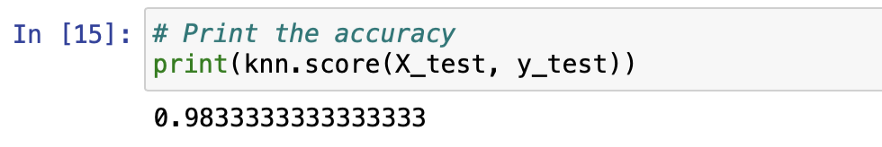y_pred = knn.predict(X_test)

number_of_equal_elements = np.sum(y_pred==y_test)
number_of_equal_elements/y_pred.shape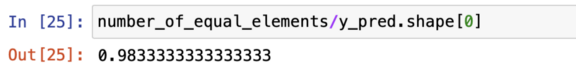### 过拟合与欠拟合

“当模型在训练数据上表现不佳时，模型对训练数据的拟合不足。这是因为模型无法捕获输入示例（特性）和目标值（标签）之间的关系。当你看到模型在训练数据上表现良好，但在评估数据上表现不佳时，该模型会过拟合你的训练数据。这是因为模型正在记忆它所看到的数据，并且无法将其推广到未看到的示例中。”（参考：https://docs.aws.amazon.com/machine-learning/latest/dg/model-fit-underfitting-vs-overfitting.html)

neighbors = np.arange(1, 9)
train_accuracy = np.empty(len(neighbors))
test_accuracy = np.empty(len(neighbors))

for i, k in enumerate(neighbors):

# 定义knn分类器
knn = KNeighborsClassifier(n_neighbors = k)

# 将分类器与训练数据相匹配
knn.fit(X_train, y_train)

# 在训练集上计算准确度
train_accuracy[i] = knn.score(X_train, y_train)

# 在测试集上计算准确度
test_accuracy[i] = knn.score(X_test, y_test)

plt.title('k-NN: Performance by Number of Neighbors')
plt.plot(neighbors, test_accuracy, label = 'Testing Accuracy')
plt.plot(neighbors, train_accuracy, label = 'Training Accuracy')
plt.legend()
plt.xlabel('# of Neighbors')
plt.ylabel('Accuracy')

plt.show()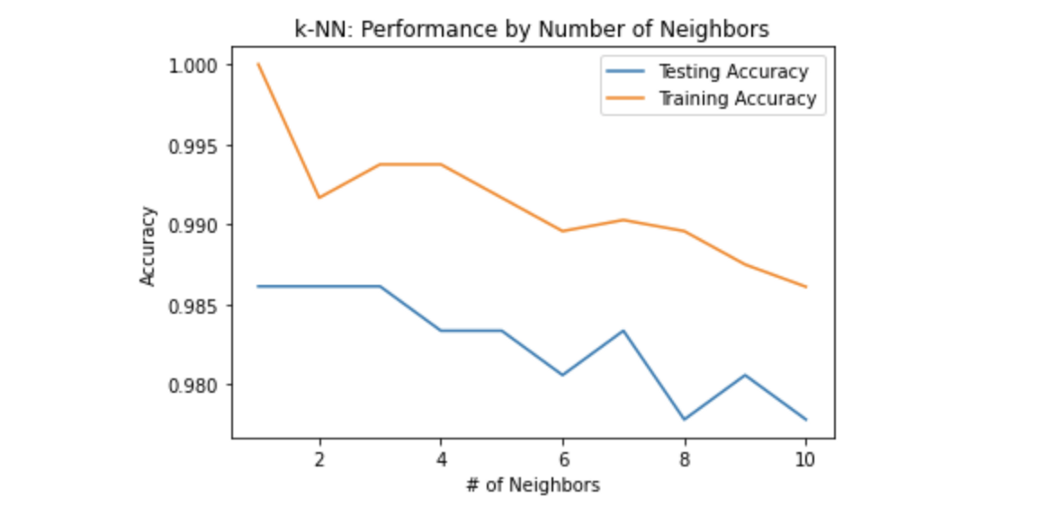knn = KNeighborsClassifier(n_neighbors = 2)
knn.fit(X_train, y_train)

print(knn.score(X_test, y_test))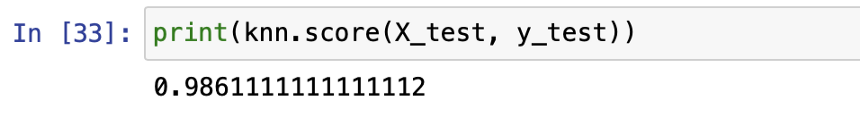### 结论

sklearn机器学习中文官方文档： http://sklearn123.com/

04-09113212-26292
08-11926
07-142274
08-31126
09-061万+
06-114887
11-2010
09-15434
11-2446
08-27130
05-02442
05-121566
09-05505
10-028535
07-15336
06-2673
09-2844万+
©️2020 CSDN 皮肤主题: 大白 设计师:CSDN官方博客